Anzeige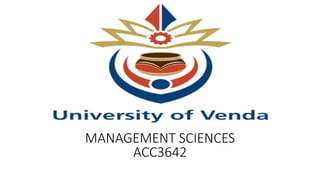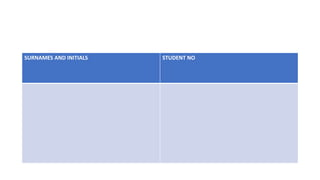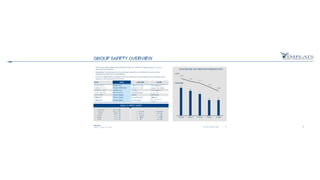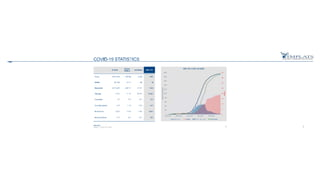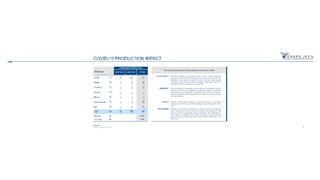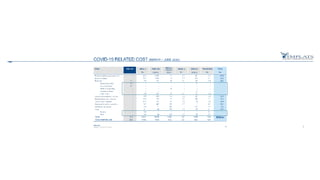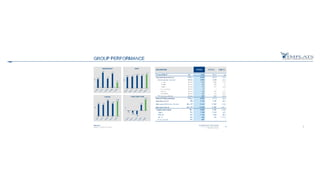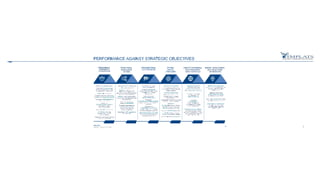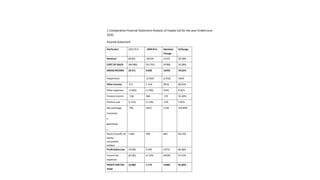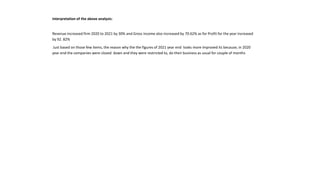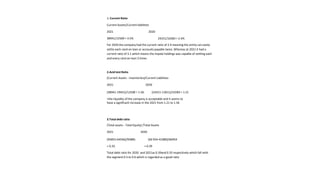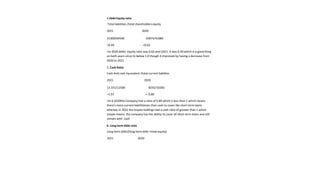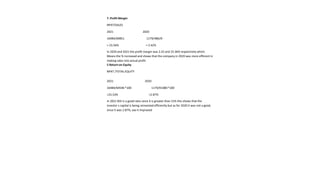1 von 13
Anzeige

### acc3642.pptx

1. MANAGEMENT SCIENCES ACC3642
2. SURNAMES AND INITIALS STUDENT NO
3. 1.Comparative Financial Statement Analysis of Impala Ltd for the year Ended June 2020 Income statement Particulars 2021 R’m 2020.R'm Absolute Change %Change Revenue 69 851 48629 21222 30.38% COST OF SALES (46 580) (41 791) (4789) 10.28% GROSSINCOME 23 271 6 838 16433 70.62% Impairment - (2 432) (2 432) 100% Other income 471 1 424 (953) 66.92% Other expenses (1 963) (1 799) (164) 8.35%. Finance income 538 368 170 31.60% Finance cost (1 155) (1 136) (19) 1.65% Net exchange transactio n gain/losse s 796 (362) 1158 319.89% Share of profit of equity accounted entities 1 082 398 684 63.22% Profit before tax 23 030 3 299 19731 85.68% Income tax expenses (6 546) (2 120) (4426) 67.61% PROFIT FOR THE YEAR 16 484 1 179 15305 92.85%
4. Interpretation of the above analysis: Revenue increased firm 2020 to 2021 by 30% and Gross income also increased by 70.62% as for Profit for the year increased by 92. 82% Just based on those few items, the reason why the the figures of 2021 year end looks more improved its because, in 2020 year end the companies were closed down and they were restricted to, do their business as usual for couple of months
5. 1. Current Ratio Current Assets/Current liabilities 2021 2020 38941/12500 = 3.5% 24251/10283 = 2.4% For 2020 the company had the current ratio of 2.4 meaning the entity can easily settle each rand on loan or accounts payable twice. Whereas at 2021 it had a current ratio of 3.1 which means the impala holdings was capable of settling each and every rand on loan 3 times 2.Acid test Ratio (Current Assets - inventories)/Current Liabilities 2021 2020 (38941-19451)/12500 = 1.56. (24251-11811)/10283 = 1.21 >the liquidity of the company is acceptable and it seems to have a significant increase in the 2021 from 1.21 to 1.56 3.Total debt ratio (Total assets - Total Equity) /Total Assets 2021 2020 (95855-64546)/95885. (66 954-41080)/66954 = 0.33. = 0.39 Total debt ratio for 2020 and 2021as 0.39and 0.33 respectively which fall with the segment 0.3 to 0.6 which is regarded as a good ratio
6. 4.Debt Equity ratio Total liabilities /total shareholders equity 2021 2020 31309/64596 25874/41080 =0.49 =0.63 >In 2020 debts equity ratio was 0.63 and i2021 it was 0.49 which is a good thing on both years since its below 1.0 though it improved by having a decrease from 2020 to 2021 5. Cash Ratio Cash And cash Equivalent /total current liabilites 2021 2020 13 331/12500 8242/10283 =1.07 = 0.80 >In 0 2020the Company had a ratio of 0.80 which is less than 1 which means there's more current liabillitiesies than cash to cover the short term loans whereas in 2021 the impala holdings had a cash ratio of greater than 1 which simple means the company has the ability to cover all short term loans and still remain with cash 6. Long term debt ratio Long-term debt/(long-term debt +total equity) 2021 2020
7. 7. Profit Margin NPAT/SALES 2021 2020 16484/69851 1179/48629 = 23.56% = 2.42% In 2020 and 2021 the profit margin was 2.42 and 23.36% respectively which. Means the % increased and shows that the company in 2020 was more efficient in making sales into actual profit 8.Return on Equity NPAT /TOTAL EQUITY 2021 2020 16484/64546 *100 1179/41080*100 =25.53% =2.87% In 2021 ROI is a good ratio since it is greater than 15% this shows that the investor s capital is being reinvested efficiently but as for 2020 it was not a good, since it was 2.87%, soo it improved
Anzeige